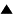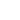Model

Metasystem Transition Theory understands knowledge as the existence in a cybernetic system of a model of some part of reality. The most immediate kind of a model is a metasystem which implements a homomorphic relation between states of two subsystems, a modeled system and a modeling system.

_________________________________________|||
S|
|          _|_ _ _ _ _ _ _ _ |           |
|                         W              |
||         || w2 = L(w1)     ||          ||
|          | w1  ______- w2  |           |
||         _|_|_ _ _ _ _ _|_ |           ||
|             |           |              |
m1|= E(w1)    ||          || m2 = E(w2)  |
||         _|_|_ _ _ _ _ _|_ |           ||
|             |?          |?             |
|          | m1  ______-m2   |           |
||         || m2 = R(m1)     ||          ||
||         _|_ _ _ _ _ _ _M_ |           ||
|________________________________________|

Figure: The Modeling Relation

Formally, a model is a system S = <W, M, E> with:

• A modeled system or world W = <W, L> with states W = {wi} and actions or laws L: W -> W . For example, W could be the set of key presses of a computer operator or the physical world, while L is the behavior of the operator or natural law;

• A modeling system M = <M, R> with internal model states, or representations M = {mj} and a set of rules, or a modeling function R: M -> M. For example, M could be a set of symbol strings or neural signals, while the rules R are the activity of a computer or a brain;

• And finally a representation function E: W -> R. For example, E could be a measurement, a perception, or an observation.

When the functions L, R, and E commute, then we have m2 = R(m1) = R(E(w1)) = E(L(w1)) = E(w2). Under these conditions S is a good model, and the modeling system M can predict the behavior of the world W. We can call S a generator of predictions about W.

However, it is possible that M is itself a model, in which case S is a meta-model. The representation function then does not generate a prediction directly, but rather generates another model, which in turn can generate predictions. We come, therefore, to the understanding of knowledge as a hierarchical structure to recursively generate predictions about the world and the self, and which in turn allow the cybernetic system to make decisions about its actions.

 HomeMetasystem Transition TheoryEpistemologyKnowledge Up Prev.Next Down